Function Repository Resource:

# PhiNumberSystem

Get a list of powers of the golden ratio which sum to a given integer

Contributed by: Wolfram Staff
 ResourceFunction["PhiNumberSystem"][n] gives the list {x1,x2,…,xk} such that ϕx1 + ϕx2 + … + ϕxk = n, where ϕ is GoldenRatio.

## Details

The list consists of nonconsecutive distinct integers that may be 0 or negative.
The input must be a nonzero integer.
ResourceFunction["PhiNumberSystem"][n] discards the sign of n.

## Examples

### Basic Examples (2)

This gives the golden ratio powers needed to represent 10:

 In:=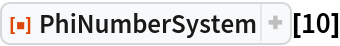Out=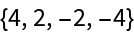Indeed, the sum of those powers of ϕ is 10:

 In:=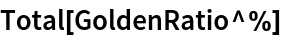Out=In:=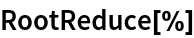Out=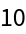### Scope (2)

Here are the base-ϕ representations of the first 20 natural numbers:

 In:=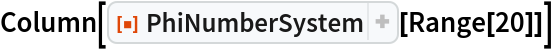Out=A large example:

 In:=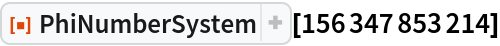Out=Check that the corresponding powers of ϕ sum to the original number:

 In:=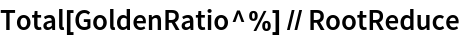Out=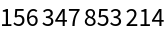### Possible Issues (1)

PhiNumberSystem[n] is left unevaluated for n=0:

 In:=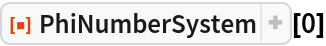Out=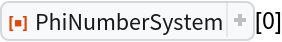### Neat Examples (1)

The sequence of the sums of the lists looks fractal:

 In:=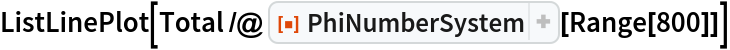Out=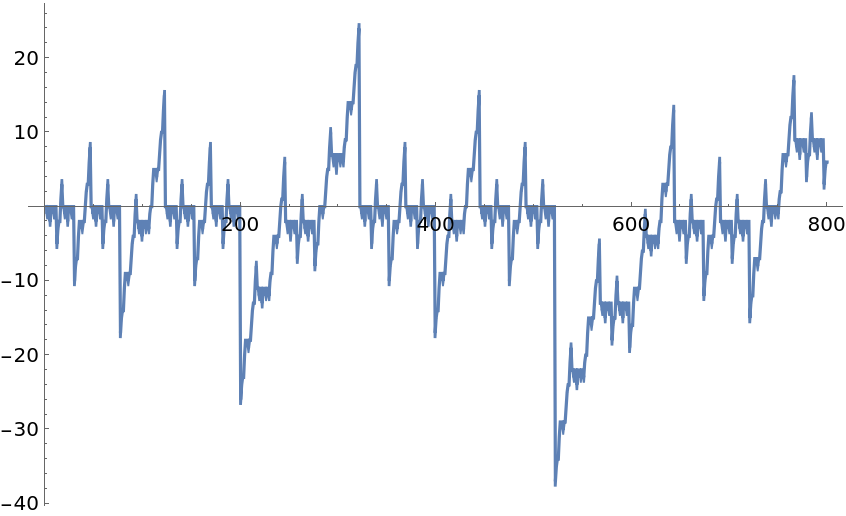George Beck

## Requirements

Wolfram Language 11.3 (March 2018) or above

## Version History

• 2.0.0 – 25 January 2021
• 1.0.0 – 26 February 2019